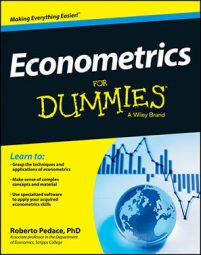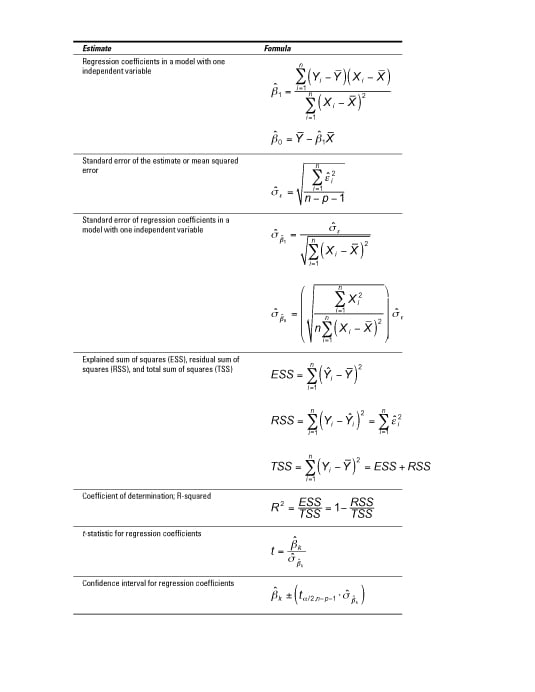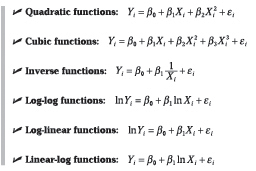##### Econometrics For DummiesYou can use the statistical tools of econometrics along with economic theory to test hypotheses of economic theories, explain economic phenomena, and derive precise quantitative estimates of the relationship between economic variables.

To accurately perform these tasks, you need econometric model-building skills, quality data, and appropriate estimation strategies. And both economic and statistical assumptions are important when using econometrics to estimate models.

## Econometric estimation and the CLRM assumptions

Econometric techniques are used to estimate economic models, which ultimately allow you to explain how various factors affect some outcome of interest or to forecast future events. The ordinary least squares (OLS) technique is the most popular method of performing regression analysis and estimating econometric models, because in standard situations (meaning the model satisfies a series of statistical assumptions) it produces optimal (the best possible) results.

The proof that OLS generates the best results is known as the Gauss-Markov theorem, but the proof requires several assumptions. These assumptions, known as the classical linear regression model (CLRM) assumptions, are the following:

• The model parameters are linear, meaning the regression coefficients don’t enter the function being estimated as exponents (although the variables can have exponents).

• The values for the independent variables are derived from a random sample of the population, and they contain variability.

• The explanatory variables don’t have perfect collinearity (that is, no independent variable can be expressed as a linear function of any other independent variables).

• The error term has zero conditional mean, meaning that the average error is zero at any specific value of the independent variable(s).

• The model has no heteroskedasticity (meaning the variance of the error is the same regardless of the independent variable’s value).

• The model has no autocorrelation (the error term doesn’t exhibit a systematic relationship over time).

If one (or more) of the CLRM assumptions isn’t met (which econometricians call failing), then OLS may not be the best estimation technique. Fortunately, econometric tools allow you to modify the OLS technique or use a completely different estimation method if the CLRM assumptions don’t hold.

## Useful formulas in econometrics

After you acquire data and choose the best econometric model for the question you want to answer, use formulas to produce the estimated output.

In some cases, you have to perform these calculations by hand (sorry). However, even if your problem allows you to use econometric software such as STATA to generate results, it’s nice to know what the computer is doing.

Here’s a look at the most common estimators from an econometric model along with the formulas used to produce them.## Econometric analysis: Looking at flexibility in models

You may want to allow your econometric model to have some flexibility, because economic relationships are rarely linear. Many situations are subject to the “law” of diminishing marginal benefits and/or increasing marginal costs, which implies that the impact of the independent variables won’t be constant (linear).

The precise functional form depends on your specific application, but the most common are as follows:## Typical problems estimating econometric models

If the CLRM doesn’t work for your data because one of its assumptions doesn’t hold, then you have to address the problem before you can finalize your analysis.

Fortunately, one of the primary contributions of econometrics is the development of techniques to address such problems or other complications with the data that make standard model estimation difficult or unreliable.

The following table lists the names of the most common estimation issues, a brief definition of each one, their consequences, typical tools used to detect them, and commonly accepted methods for resolving each problem.

Problem Definition Consequences Detection Solution
High multicollinearity Two or more independent variables in a regression model exhibit
a close linear relationship.
Large standard errors and insignificant
t-statistics
Coefficient estimates sensitive to minor changes in model
specification
Nonsensical coefficient signs and magnitudes
Pairwise correlation coefficients
Variance inflation factor (VIF)
2. Re-specify the model.
3. Drop redundant variables.
Heteroskedasticity The variance of the error term changes in response to a change
in the value of the independent variables.
Inefficient coefficient estimates
Biased standard errors
Unreliable hypothesis tests
Park test
Goldfeld-Quandt test
Breusch-Pagan test
White test
1. Weighted least squares (WLS)
2. Robust standard errors
Autocorrelation An identifiable relationship (positive or negative) exists
between the values of the error in one period and the values of the
error in another period.
Inefficient coefficient estimates
Biased standard errors
Unreliable hypothesis tests
Geary or runs test
Durbin-Watson test
Breusch-Godfrey test
1. Cochrane-Orcutt transformation
2. Prais-Winsten transformation
3. Newey-West robust standard errors